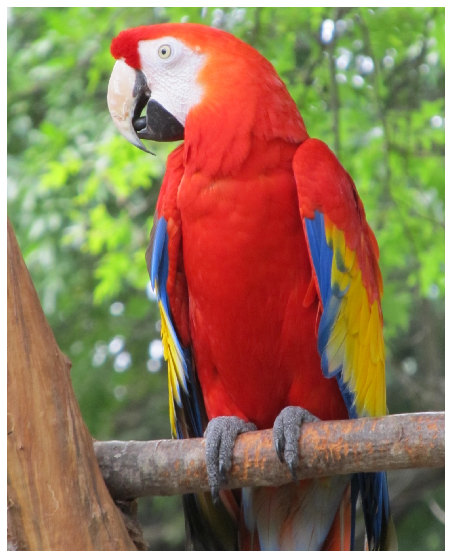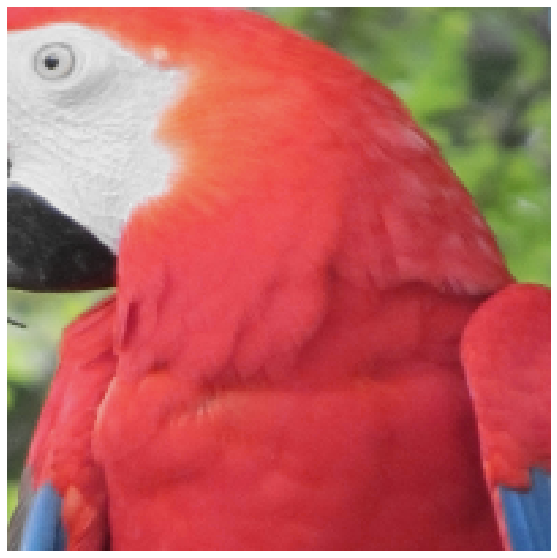# Debugging an augmentation pipeline with ReplayCompose¶

An augmentation pipeline has a lot of randomness inside it. It applies augmentations with some probabilities, and it samples parameters for those augmentations (such as a rotation angle or a level of changing brightness) from a random distribution.

It could be very useful for debugging purposes to see which augmentations were applied to the image and look at the parameters of those augmentations.

`ReplayCompose` tracks augmentation parameters. You can inspect those parameters or reapply them to another image.

### Import the required libraries¶

``````import random

import cv2
import matplotlib.pyplot as plt
import albumentations as A
``````

### Define the visualization function¶

``````def visualize(image):
plt.figure(figsize=(10, 10))
plt.axis('off')
plt.imshow(image)
``````

### Load an image from the disk¶

``````image = cv2.imread('images/parrot.jpg')
image = cv2.cvtColor(image, cv2.COLOR_BGR2RGB)
visualize(image)
``````### Declare an augmentation pipeline using `ReplayCompose`¶

``````transform = A.ReplayCompose([
A.Resize(512, 512, always_apply=True),
A.RandomCrop(200, 200, always_apply=True),
A.OneOf([
A.RGBShift(),
A.HueSaturationValue()
]),
])
``````

We fix the random seed for visualization purposes, so the augmentation will always produce the same result. In a real computer vision pipeline, you shouldn't fix the random seed before applying a transform to the image because, in that case, the pipeline will always output the same image. The purpose of image augmentation is to use different transformations each time.

``````random.seed(7)
data = transform(image=image)
visualize(data['image'])
```````data['replay']` contains information about augmentations that ere applied to the image. If `applied` is `True`, then the augmentation was applied to the image. `params` contains information about parameters that were used to transform the image.

``````data['replay']
``````
``````{'__class_fullname__': 'albumentations.core.composition.ReplayCompose',
'params': None,
'transforms': [{'__class_fullname__': 'albumentations.augmentations.transforms.Resize',
'always_apply': True,
'p': 1,
'height': 512,
'width': 512,
'interpolation': 1,
'params': {},
'applied': True},
{'__class_fullname__': 'albumentations.augmentations.transforms.RandomCrop',
'always_apply': True,
'p': 1.0,
'height': 200,
'width': 200,
'params': {'h_start': 0.07243628666754276, 'w_start': 0.5358820043066892},
'applied': True},
{'__class_fullname__': 'albumentations.core.composition.OneOf',
'params': None,
'transforms': [{'__class_fullname__': 'albumentations.augmentations.transforms.RGBShift',
'always_apply': False,
'p': 0.5,
'r_shift_limit': (-20, 20),
'g_shift_limit': (-20, 20),
'b_shift_limit': (-20, 20),
'params': None,
'applied': False},
{'__class_fullname__': 'albumentations.augmentations.transforms.HueSaturationValue',
'always_apply': False,
'p': 0.5,
'hue_shift_limit': (-20, 20),
'sat_shift_limit': (-30, 30),
'val_shift_limit': (-20, 20),
'params': {'hue_shift': -2.654172653504567,
'sat_shift': -25.808674585522866,
'val_shift': -16.371479466245397},
'applied': True}],
'applied': True}],
'applied': True}```
```

### Using `ReplayCompose.replay` to apply the same augmentations to another image¶

To apply the same set of augmentations to a new target, you can use the `ReplayCompose.replay` function.

``````image2 = cv2.imread('images/image_2.jpg')